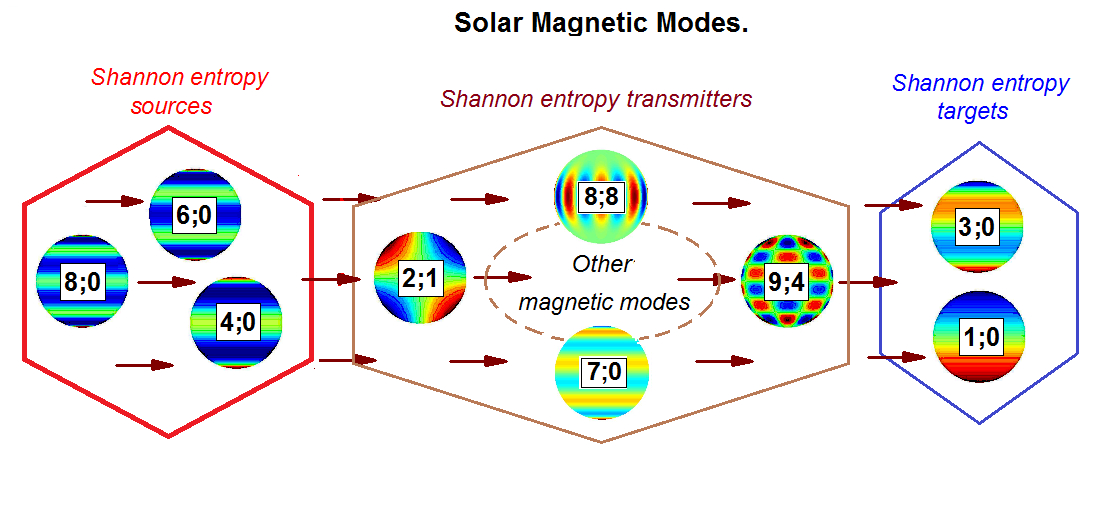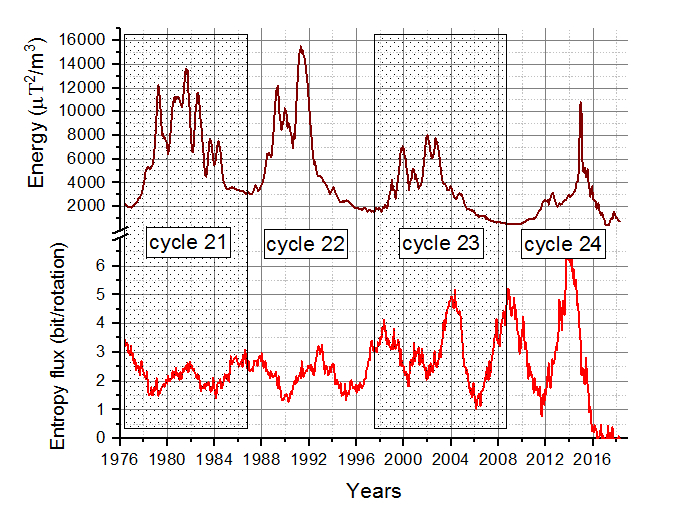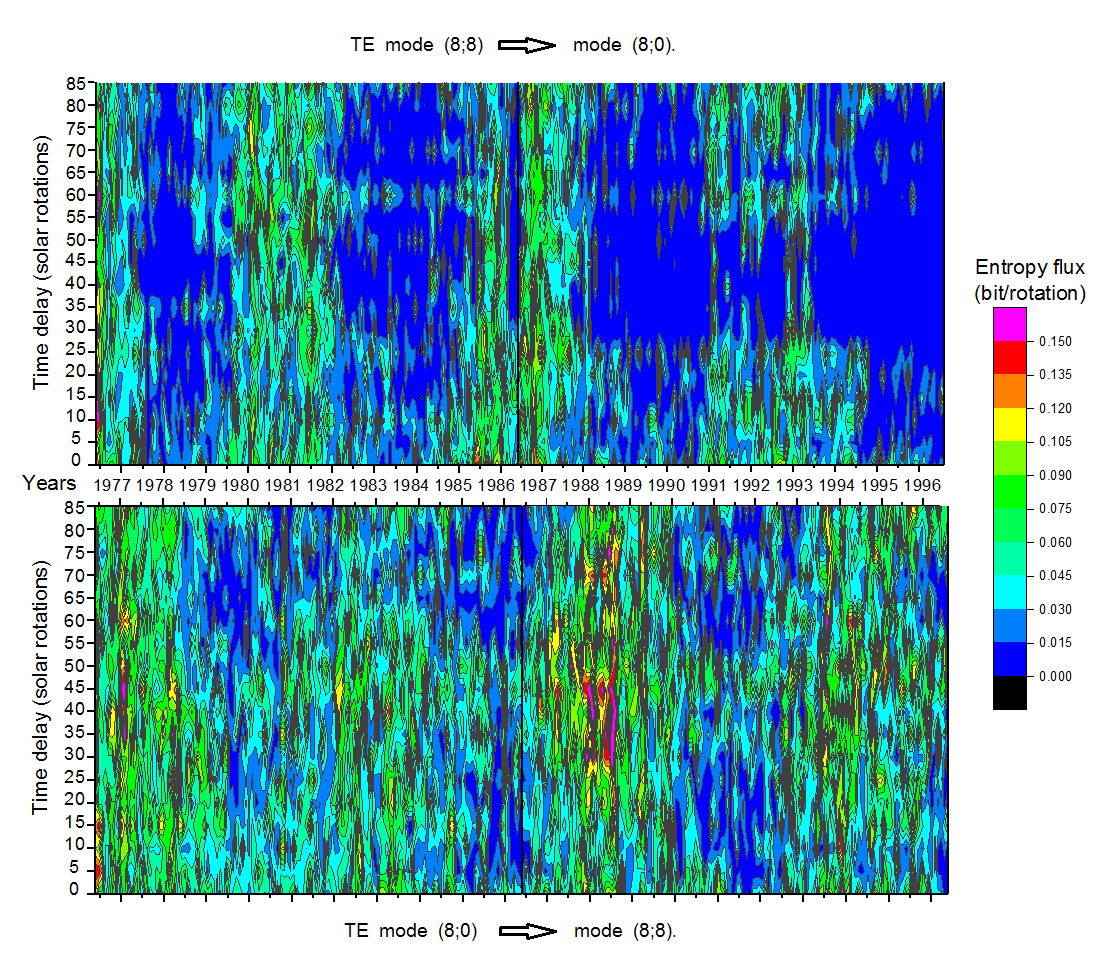# 132. Specifics Of Shannon Entropy Transferring Between Solar Magnetic Modes

Contributed by V. P. Mikhaylutsa. Posted on October 22, 2019

V. P. Mikhaylutsa
Indivdual researcher

Application of Shannon’s entropy technique in solar physics opens up new facts. This work is the continuation of the research, first results of which have been published by Mikhaylutsa. In our previous work we have used and studied the original Stanford magnetic field harmonic coefficients for 4 solar cycles (21–24) by means of applying the Shannon entropy transfer technique to the time-series of magnetic mode energies. We should refer the readers – who are not familiar with how and what solar parameters are used to calculate this entropy – to the article Ref. Here, we remind the scheme of sequential transfer of the Shannon’s entropy illustrated in Figure 1. Three main groups of modes are allocated: the entropy sources, the entropy transmitters, and the entropy targets (or receivers). The entropy sources (modes (8; 0), (6; 0) and (4; 0)) have no net entropy flux input; the entropy targets (modes (1; 0) and (3; 0)) have no net entropy flux output. The entropy transmitters have inputs and outputs, and are situated between sources and targets. Mode (2; 1) is the first in the chain of the entropy transmitters; mode (9; 4) is the last one. Below, some new details of Shannon entropy transferring between magnetic modes of the Sun are presented and discussed.Figure 1| Sequence of the transferring of the net Shannon entropy between solar magnetic modes. Arrows indicate directions of net entropy flux. Only magnetic modes with special roles are shown, others modes are hidden inside the dashed oval. The colors (from blue to red) illustrate magnetic polarity structure.

Mode (2; 1) is the principal in the modulated transferring of the Shannon entropy into other solar modes. Modulation of the Shannon entropy transferring between magnetic modes is independent on the mode’s intensity and has not been revealed for all modes. The mode (2; 1) – “tesseral-quadrupole” – is associated with the largest number of modes to which the modulated Shannon entropy has been transmitted. The total entropy flux from the mode (2; 1) is shown by red curve at the bottom of Figure 2. The modulation of the flux is well seen. Obviously, each beginning of the solar cycle coincides with the maximum of the Shannon entropy flux from the mode (2; 1). Period of the modulation is about 5.4 years. The increasing amplitudes of modulation is due to statistical problems.This tesseral-quadrupole magnetic mode is involved in the synchronization of the global magnetic cycles.Figure 2| Cycles of the magnetic energy density of the Sun (above) and the total flux of Shannon entropy from the mode (2; 1) (below). Solar cycles are highlighted by retouching. The Shannon entropy flux is measured in (bit/solar rotation), where bit is the smallest unit of measurement used to quantify informational data.

Shannon entropy transfer from/to the mode can occur with phase shifts between counter modulated flows. As a result, it leads to a final net entropy flux with a periodical change in direction (flow circulation). Physically, this means that such modes are interdependent. Dependence may be direct or indirect, that does not matter in our case. Mode (8; 8) is the principal in the processes of circulation of entropy transfer between solar magnetic modes. As an example, in two-dimensional Figure 3. the Shannon entropy exchanges between this mode and zonal mode (8; 0) are shown. Both maps in the Figure 3. demonstrate periodic modulation of the informational entropy flow (green and blue vertical bands). The phase shift between bands can be seen. So, the informational entropy is circulating. More intense yellow-green colors of the lower map indicate that the information flow from the mode (8; 0) dominates over the flow from mode (8; 8). All characteristics are preserved in bulk, regardless of the delay-time between the source and target time-series. It means that the dependence between current state of the
source-mode cell and all future states of target-mode cells has been kept at least up to 90 solar rotations. This mysterious situation can be understood if we assume that there is a special process on the Sun. That process periodically (twice per sunspot cycle) generates a cluster of interconnected magnetic modes, which will evolve in a predetermined manner (“template”). Identifying this “template” is a good goal for researchers. Probably, such “template” could exists inside “entropy-transferring-family” modes.Figure 3| Two-dimensional maps of the Shannon entropy transferring from the mode (8; 8) to the mode (8; 0) – upper picture, and in back direction – bottom picture. Horizontal axis – is the time in years (from 1976 up to 1997), vertical axis – is the delay-time between the time-series (in solar rotations). The color scale of the entropy flux is shown at the right side of the figure.

### References

 Kakad, B., Kakad, A., & Ramesh, D. S. 2017, Solar Phys., 292, 12
 Wing, S., & Johnson, J. R. 2019, Entropy, 21, 140
 Mikhaylusta, V. P. 2019, J. Astrophys. Astron., 40, 22